# C#中如何利用操作符重载和转换操作符

+关注继续查看

## 操作符重载

namespace DoNet.Seven.ConsoleApplicationTest
{
class Program
{
static void Main(string[] args)
{
rational r1 = new rational(10);
rational r2 = new rational(5);
rational r3= r1 + r2;
Console.WriteLine(r3.Value);

}
}
//有理数
public sealed class rational
{
private int _value = 0;

public int Value
{
get { return _value; }
set { _value = value; }
}
public rational(int value)
{
this._value = value;
}

public rational()
{

}
public static rational operator+(rational num1,rational num2)
{
rational result = new rational(num1.Value+num2.Value);
return result;
}

}
}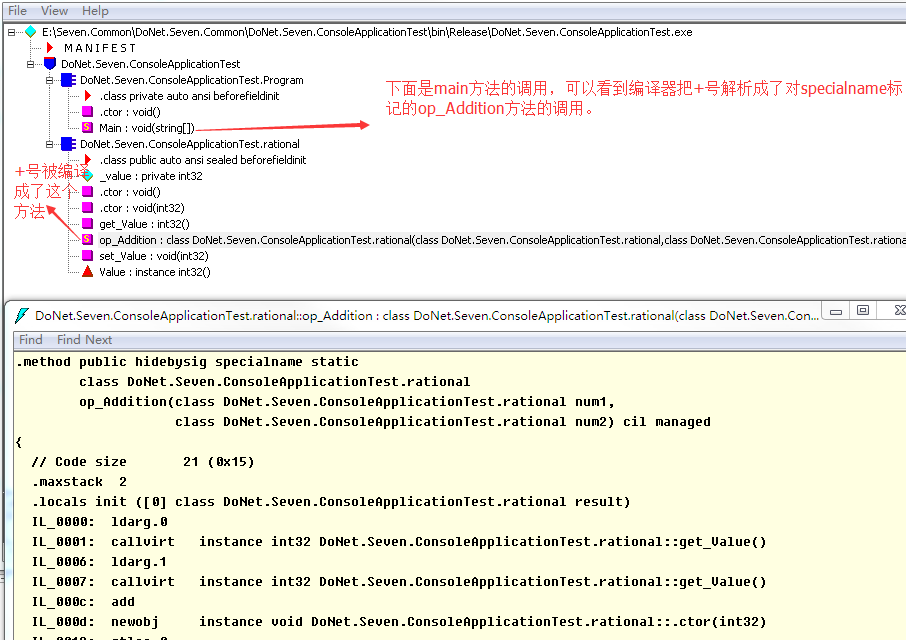1、首先CLR规范要求操作符重载方法必须是public和static方法。另外，C#编译器要求操作符重载方法至少有一个参数的类型与当前定义的这个方法的类型相同。之所以这样做，

3、对于其它操作符编译之后对应的方法如下表所示（左边是一元操作符，右边是二元操作符）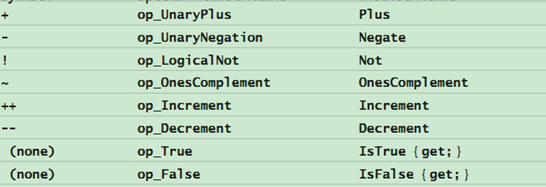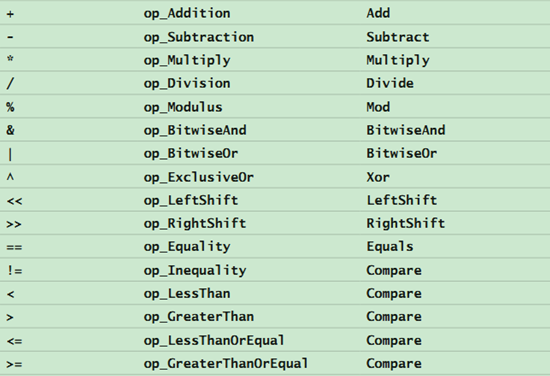## 转换操作符

int转换为string，当然也可以转换为其它类型。就像上面的rational一样，如果能将一个int或者double转换为一个rational，就会很方便，反之亦然。

 //有理数
public sealed class rational
{
private int _value = 0;

public int Value
{
get { return _value; }
set { _value = value; }
}
public rational(int value)
{
this._value = value;
}

public rational(double value)
{
this._value =(int)value;
}
public rational()
{

}

public int ToInt()
{
return _value;
}
public double ToDouble()
{
return (double)_value;
}
public static rational operator+(rational num1,rational num2)
{
rational result = new rational(num1.Value+num2.Value);
return result;
}

}

1、调用这些构造器和方法，开发人员可以很方便的将int和double对象转换成rational对象，这将给编程工作带来很多方便。设计类型时，应该认真考虑类型需要支持的转换构造器和方法。

2、int i=10;long j=i;这样的代码我们经常会看到，那么从int类型到long类型的转换为什么就可以隐士的进行呢？这就涉及到了我们的转换操作符，下面我们也为rational定义几个转换操作符。

namespace DoNet.Seven.ConsoleApplicationTest
{
class Program
{
static void Main(string[] args)
{
int n = 10;
rational r1 = n;
double d=(double)r1;

Console.WriteLine(r1.Value);
Console.WriteLine(d.ToString());

}
}
//有理数
public sealed class rational
{
private int _value = 0;

public int Value
{
get { return _value; }
set { _value = value; }
}
public rational(int value)
{
this._value = value;
}

public rational(double value)
{
this._value =(int)value;
}
public rational()
{

}

public int ToInt()
{
return _value;
}
public double ToDouble()
{
return (double)_value;
}
public static rational operator+(rational num1,rational num2)
{
rational result = new rational(num1.Value+num2.Value);
return result;
}

public static implicit operator rational(int value)
{
return new rational(value);
}
public static implicit operator rational(double value)
{
return new rational(value);
}
public static explicit operator int(rational value)
{
return value.ToInt();
}
public static explicit operator double(rational value)
{
return value.ToDouble();
}

}
}

C#编译器检测到代码中的转型，并内部生成IL代码来调用rational类型定义的转换操作符方法，如果用反编译器看的话可以发现，转换操作符方法会生成下面这样的代码：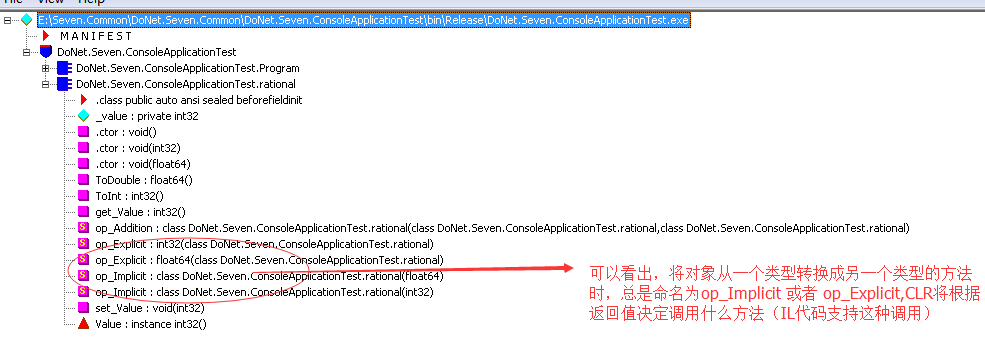## 结论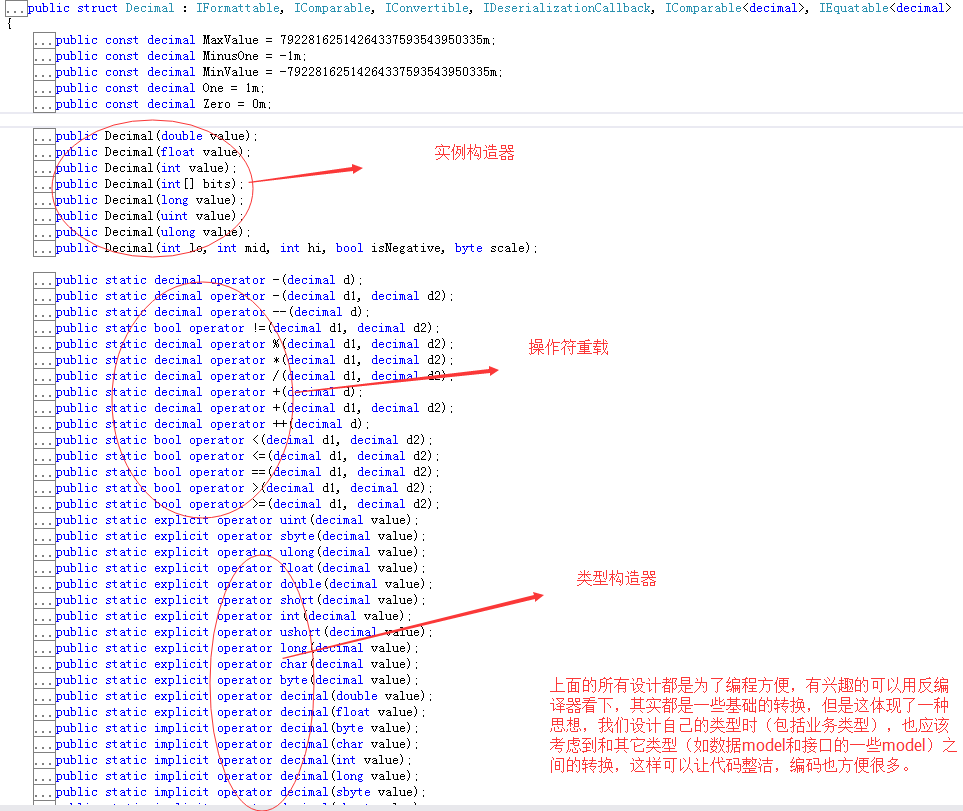【C#基础】C# 运算符总结

11 023 0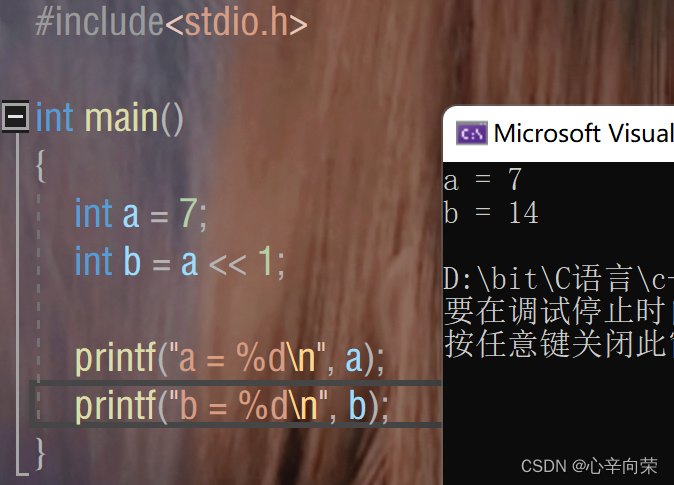【C】操作符归纳+表达式运算原理
【C】操作符归纳+表达式运算原理
129 031 0（C语言基础）操作符详解2（数据在内存中的存储规则）以及字符串的倒置（详解）
（C语言基础）操作符详解2（数据在内存中的存储规则）以及字符串的倒置（详解）
34 0＜C++＞运算符重载进阶之左移运算符，输出成员属性一步到位
＜C++＞运算符重载进阶之左移运算符，输出成员属性一步到位
76 0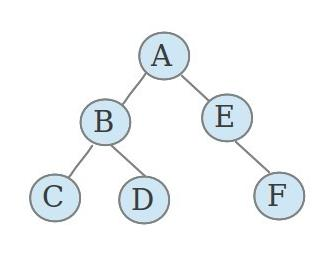C#表达式树 创建、生成、使用、lambd a转成表达式树~表达式树的知识详解
C#表达式树 创建、生成、使用、lambd a转成表达式树~表达式树的知识详解
158 0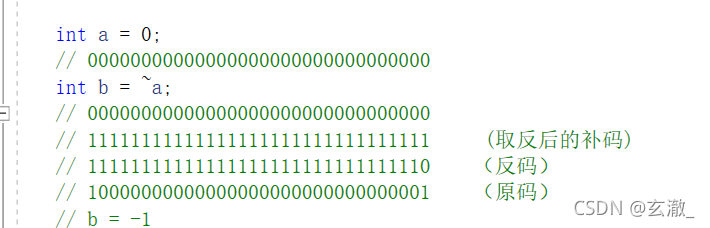【C语言操作符多图详解】——“讲述你所未知的操作符细节”(下)
【C语言操作符多图详解】——“讲述你所未知的操作符细节”(下)
36 0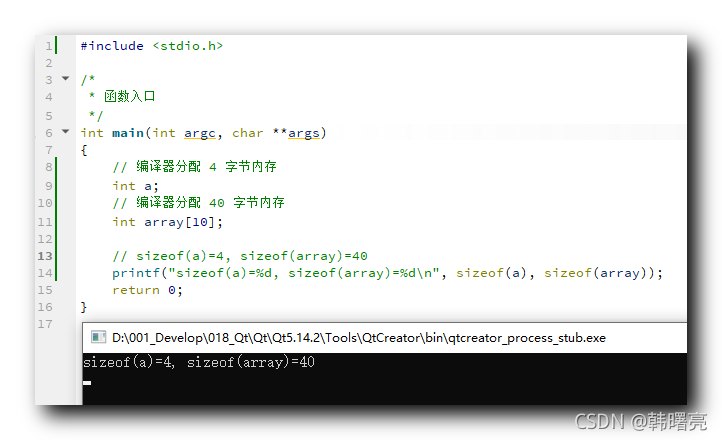【C 语言】数据类型本质 ( sizeof 函数 | 数据类型大小 )
【C 语言】数据类型本质 ( sizeof 函数 | 数据类型大小 )
105 0

Python语言基础 - 列表、元组、字典、集合5306220